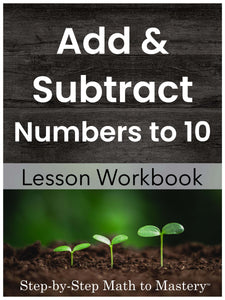# Add and Subtract Numbers to 10 Lesson Workbook

• \$15.00
Unit price per
Shipping calculated at checkout.

## Addition & Subtraction Numbers to 10 (Sums within 20)

### 79 Step-by-Step Math Lessons | Special Education Math Intervention | 1st Grade Math Level

This Addition and Subtraction to 10 lesson workbook includes an example IEP goal, step-by-step math lessons, reviews and assessments. This evidence-based math intervention is tied to first grade standards and is great for special education math goals and tier 2 small group math interventions (RTI).

These lessons were created for students who need a solid strategy they can rely on for addition and subtraction. Ten frames, number lines, pictures, and even fingers can be helpful when illustrating the concept of addition, but there’s a time when students won’t be able to use these. What can they use if they have difficulty memorizing facts? Tap the dots!

These sequential lessons are easy to teach, with enough material for 24 weeks of instruction!

### Click Here to Preview

79 Lessons
39 Reviews
3 Tests

214 Pages
24 Weeks of Instruction

## Students Will Be Able To:

• Add numbers to 9 using the count on strategy with touch dots
• Write a related fact with the addends in a different order (commutative property)
• Memorize the doubles facts (1+1, 2+2, 3+3, etc.)
• Write doubles +1 facts
• Subtract numbers to 9 using the count back strategy with touch dots
• Write related subtraction facts
• Subtract using memorized doubles facts (10 - 5,  8 - 4,  6 - 3, etc.)
• Subtract “neighbor” numbers (9 - 8, 6 - 5,  3 - 2)

## Lessons Are Tied to These Standards:

Relate counting to addition and subtraction.  (e.g., by counting on 2 to add 2)

Add and subtract within 20, demonstrating fluency for addition and subtraction within 10.  Use strategies such as counting on; making ten; decomposing a number leading to a ten; using the relationship between addition and subtraction; and creating equivalent but easier or known sums.

Apply properties of operations as strategies to add and subtract.  (These lessons teach the commutative property of addition through related facts.)

Fluently add and subtract within 20 using mental strategies.  By end of Grade 2, know from memory all sums of two one-digit numbers.

## Teacher Feedback:

❤️ “I absolutely love this resource! I love how the concepts are broken down and give the students a hands-on strategy. I love how many practice pages per concept as well. I've been looking for a good math intervention program for my students and this one is awesome! My students are making so many gains! I liked this so much I got the entire program!”-Jacqueline R.

❤️ “I love how easy these are to use and the growth my students have shown with these resources!” -Angela J.

❤️ “Thanks so much! Easy to prep and follow. My go-to!” -Megan A.

❤️ “Very helpful when teaching my learning-disabled students. Thank you.” -Growing Special Minds

### Not sure where to start?

Watch a video overview of Step-by-Step Math to Mastery or read the transcript here.

### Step-by-Step Math to Mastery™ Resources:

Make math easier to understand.

• Help students over math hurdles with clear, sequential, scaffolded lessons.
• Prevent overwhelm. Build student confidence.

Make math easier to teach.

• Save hours of planning and piecing together materials.
• Paraeducators can deliver quality instruction independently. Open & teach.

### Reasons You'll Love Step-by-Step Math to Mastery™ Resources

• Boosts student confidence and progress
• Time-saving: print and teach
• Easy prep: only black ink is needed
• Easy to teach, paraeducator-friendly
• Example IEP goals and shorter term objectives
• Consistent & predictable format
• Lots of practice repetitions
• Scaffolded with structured workspaces
• Fewer problems on a page, white space, minimal visual clutter
• Tied to standards
• Explicit/Direct instruction
• Systematic & sequential
• Mastery approach--teaching one topic at a time, one strategy at a time
• Lessons have "I Can" statements, model problems, guided practice, & independent practice
• Each workbook can be used individually as a stand-alone intervention for that skill or they can be used together, taking students from the basics of number sense and addition in first grade all the way to dividing fractions and decimals in fifth grade.
• Can also be used with older students (middle & high school) to help fill the gaps in their learning.